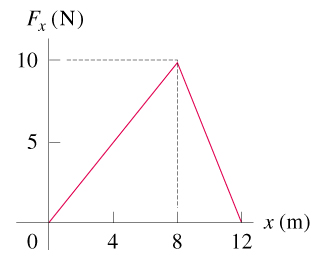# Problem: A child applies a force F parallel to the x -axis to a 10.0-kg sled moving on the frozen surface of a small pond. As the child controls the speed of the sled, the x -component of the force she applies varies with the x -coordinate of the sled as shown in the figure .Calculate the work done by the force F when the sled moves from x=0 to x=8.0 m.Calculate the work done by the force F when the sled moves from x=8.0 m to x =12.0 m.Calculate the work done by the force F when the sled moves from x=0 to x =12.0 m. .

###### Problem Details

A child applies a force F parallel to the x -axis to a 10.0-kg sled moving on the frozen surface of a small pond. As the child controls the speed of the sled, the x -component of the force she applies varies with the x -coordinate of the sled as shown in the figure.

Calculate the work done by the force F when the sled moves from x=0 to x=8.0 m.

Calculate the work done by the force F when the sled moves from x=8.0 m to x =12.0 m.

Calculate the work done by the force F when the sled moves from x=0 to x =12.0 m.
.